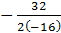### Sample Problem

You throw a ball into the air from a height of 5 feet with an initial vertical velocity of 32 feet per second. Use the vertical motion model, h = -16t2 + vt + s where v is the initial velocity in feet/second and s is the height in feet, to calculate the maximum height of the ball. Round your answer to the nearest tenth if necessary.

Maximum height: feet

#### Solution

h = -16t2 + vt + s

h = -16t2 + 32t + 5

x-coordinate of vertex:= 1

y-coordinate of vertex: h = -16(1)2 +32(1) + 5 = 21